RELATED is one of the most commonly used DAX functions. You use RELATED when you are scanning a table, and within that row context you want to access rows in related tables. RELATEDTABLE is the companion of RELATED, and it is used to traverse relationships in the opposite direction. When learning DAX, it is easy to get confused and use RELATED when it is not necessary, or to forget about RELATEDTABLE. In this article, we describe the most common uses of the two functions, along with common misperceptions.

When you have a row context open on a table, you can access the value of any column in the table being iterated. If you are not familiar with the row context, you can learn more about it here: Row context in DAX. You can access any column in the table being iterated, but you cannot access columns in related tables. We use the following model as an example.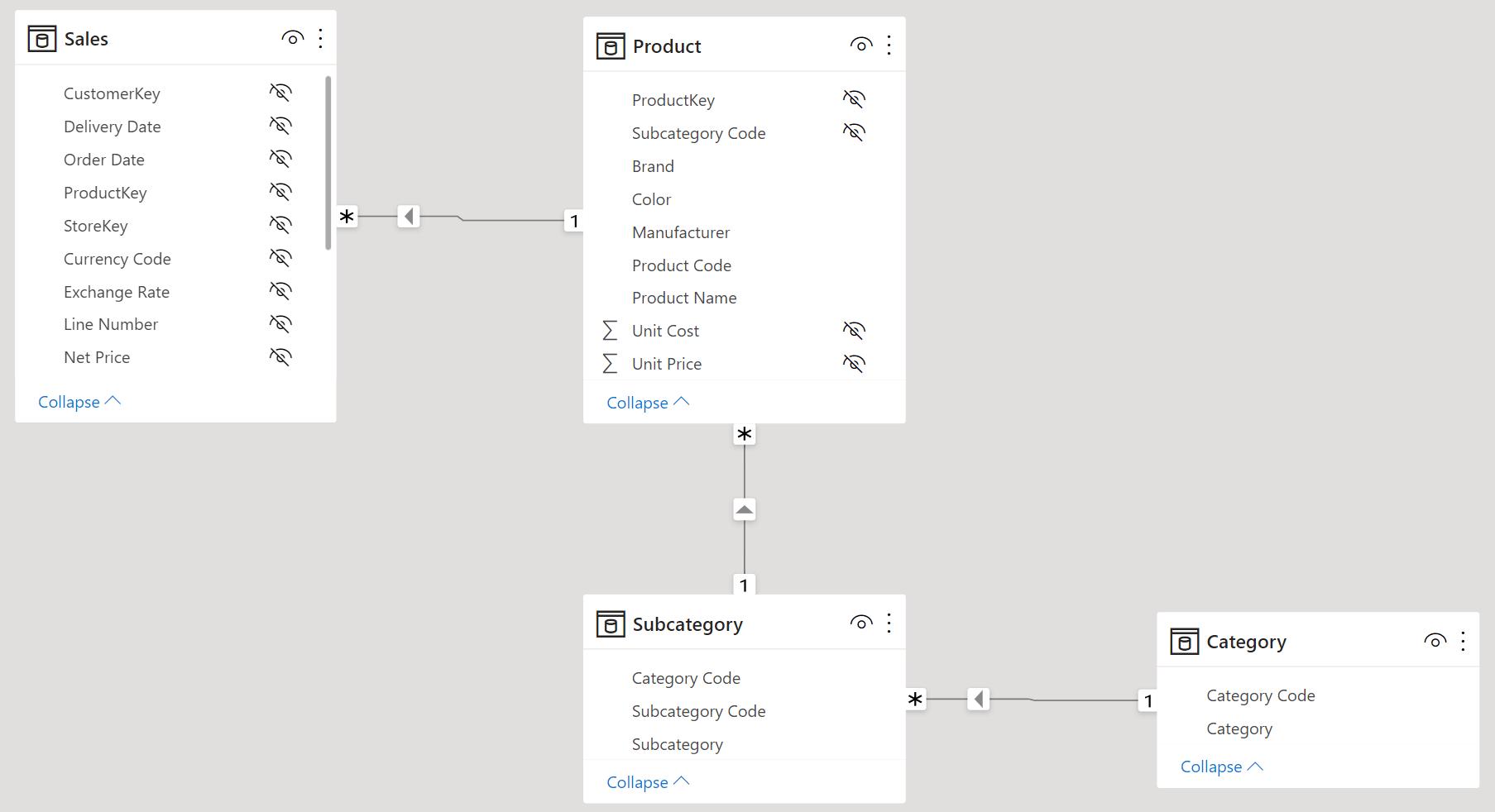There is a chain of relationships starting from Sales and reaching Product first, then Subcategory, and finally Category. All the relationships are many-to-one relationships, meaning that given an individual sale, we look at only one product, one subcategory, and one category related to that given transaction.

Despite the relationships being in place, a calculated column in Sales cannot reference directly columns in Product. This calculated column would produce an error:

Calculated Column in the Sales table
```Discount = Product[Unit Price] - Sales[Net Price]
```

Indeed, the row context on Sales does not let you reference columns in Product, although the relationship is in place. By default, the row context does not propagate through relationships. Accessing columns in related tables requires you to use the RELATED function. This code is what we need:

Calculated Column in the Sales table
```Discount = RELATED ( Product[Unit Price] ) - Sales[Net Price]
```

RELATED works because the row context is iterating the table on the many-side of a relationship. Because of this, in Product there is only one row related to the transaction being iterated. Therefore, RELATED returns the value of the column in that unique row.

RELATED can traverse chains of relationships, as long as they all are in the same many-to-one direction. For example, if you needed to access the Category[Category] column, which is far from the Sales table, you could simply use RELATED again:

Calculated Column in the Sales table
```QuantityForAudio =
IF (
RELATED ( Category[Category] ) = "Audio",
Sales[Quantity],
0
)
```

One important note about RELATED is that RELATED requires a regular relationship to work. RELATED does not work if any of the involved relationships is a limited relationship. For example, look at the following model, where we added a copy of Product, named Product DQ, which works in DirectQuery mode.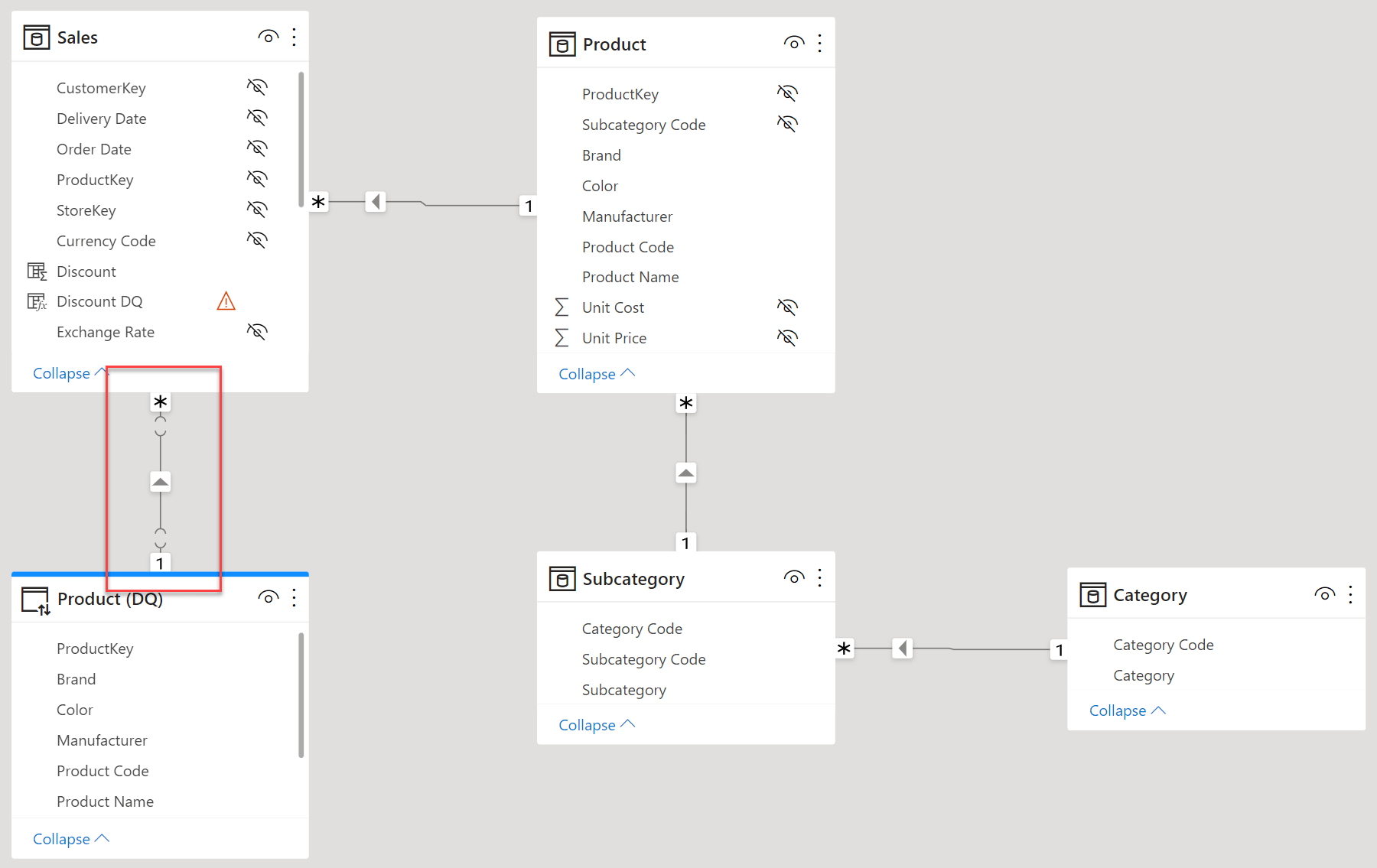Because Product (DQ) is on a separate data island, the relationship linking Sales and Product (DQ) is limited. The Discount DQ column uses the same code as Discount, but it is using the Product (DQ) table instead of Product, and it produces an error:

Calculated Column in the Sales table
```Discount DQ = RELATED ( 'Product (DQ)'[Unit Price] ) - Sales[Net Price]
```

The problem here is not that RELATED does not work over DirectQuery. RELATED does not work because the relationship crosses the borders of a data island, which makes it a limited relationship. If both Sales and Product were in DirectQuery, then RELATED would work just fine. It is the mixing of different data islands that prevents the relationship from being regular, and thus prevents RELATED from working.

RELATED works from the many-side of a relationship towards the one-side. When you need to traverse the relationship in the opposite direction, you can use RELATEDTABLE. When the row context is iterating the one-side of a relationship, there are potentially many rows in the many-side that relate to the current row. Hence, RELATED would not be an option because RELATED returns a single value. In that case, you can use RELATEDTABLE to retrieve a table with all the rows in the related table that reference the row being iterated.

For example, the following calculated column in Category counts the number of transactions for each category:

Calculated Column in the Category table
```Num Transactions = COUNTROWS ( RELATEDTABLE ( Sales ) )
```

The result is the number of rows in Sales that are related to each category.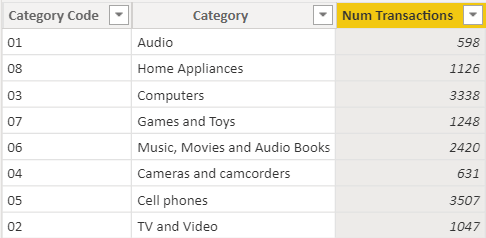It is worth mentioning that RELATEDTABLE is not a real function. RELATEDTABLE is an alias for CALCULATETABLE, added to the DAX language to be the companion of RELATED and to increase readability. RELATEDTABLE returns a table with all the related rows by leveraging context transition. If you are not familiar with the concept context transition, you may find helpful to read Understanding context transition in DAX.

Knowing that RELATEDTABLE is actually an alias for CALCULATETABLE helps us understand why RELATEDTABLE uses all the existing row contexts to propagate relationships. As an educational exercise, look at the following calculated column, still in Category:

Calculated Column in the Category table
```Avg Prod Transactions =
AVERAGEX (
RELATEDTABLE ( 'Product' ),
COUNTROWS ( RELATEDTABLE ( Sales ) )
)
```

There are two instances of RELATEDTABLE. The first instance, over Product, is executed in the row context iterating over the Category table. Therefore, it returns the products that belong to the current category. The second RELATEDTABLE, over Sales, is executed in a row context that is iterating over Product. Therefore, when the second RELATEDTABLE is executed, there are actually two row contexts active: one over Category and one over Product. The inner row context (the row context over Product) is more restrictive than the outer row context (the row context over Category). Therefore, the calculated column computes the average number of transactions per product, for all the products in the current category.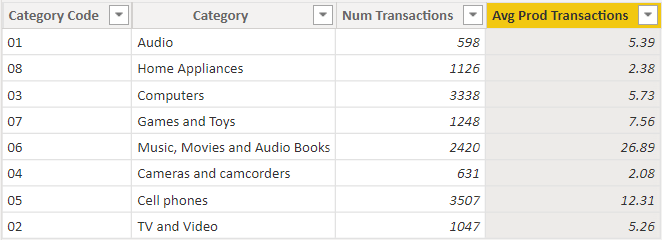In this example, the outer row context was always less restrictive than the inner row context. Indeed, filtering one individual product is always more restrictive than filtering all the products in one category. You may however face situations with nested row contexts, where the inner row context is not restricting the outer row context. For example, look at the following measure that computes the average yearly sales of a category:

Calculated Column in the Category table
```AvgYearlyTransactions =
AVERAGEX (
ALLNOBLANKROW ( 'Date'[Year] ),
COUNTROWS ( RELATEDTABLE ( Sales ) )
)
```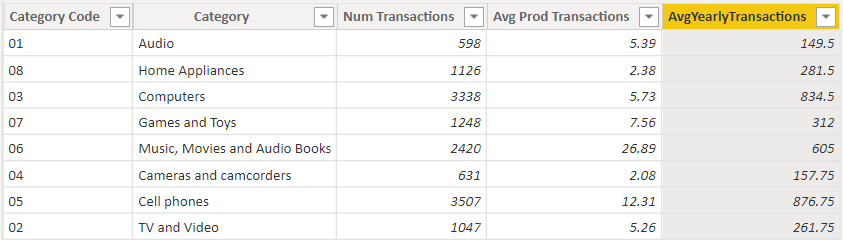When RELATEDTABLE is executed, there are two row contexts: one over the current row in Category and one over the Date[Year] column. Both are used to propagate their filter to Sales. The reason for this behavior is that the context transition induced by RELATEDTABLE ( Sales ) generates a filter context with all the existing row contexts being transformed into a filter context.

RELATEDTABLE being an alias for CALCULATETABLE also explains why RELATEDTABLE is able to traverse limited relationships, whereas RELATED is not. Demonstrating this behavior is a bit more complex, because we cannot use calculated columns in DirectQuery tables if RELATEDTABLE is involved. Regardless, look at the following measure that computes the number of transactions of the top 10 products in order of sales amount:

Measure in the Sales table
```Best Product Sales (DQ) :=
VAR BestProducts = TOPN ( 10,'Product (DQ)', [Sales Amount] )
VAR TransOfBestProducts =
SUMX (
BestProducts,
COUNTROWS ( RELATEDTABLE ( Sales ) )
)
RETURN
TransOfBestProducts
```

When the TransOfBestProducts variable is being computed, it relies on RELATEDTABLE to retrieve the rows in Sales that pertain to the product being iterated. The relationship between Product (DQ) and Sales is a limited relationship, and yet the measure works.## Conclusion

RELATED and RELATEDTABLE are simple functions, that are useful to navigate through relationships within a row context. To go a bit farther on the topic of RELATED and RELATEDTABLE, there is one challenging scenario that is when we need to handle inactive relationships. Indeed, RELATED follows the currently active relationship and making it follow an inactive relationship proves to be much harder than expected. The topic is very advanced, definitely too advanced to be covered in this introductory article. We suggest that the interested (and patient) readers take a look at the following article, which covers interactions between USERELATIONSHIP and RELATED: USERELATIONSHIP in calculated columns and Expanded tables in DAX. These go deeper on the topic of table expansion, restricted to how RELATED works.

RELATEDTABLEContext transition

Returns the related tables filtered so that it only includes the related rows.

`RELATEDTABLE ( <Table> )`

CALCULATETABLEContext transition

Evaluates a table expression in a context modified by filters.

`CALCULATETABLE ( <Table> [, <Filter> [, <Filter> [, … ] ] ] )`

USERELATIONSHIPCALCULATE modifier

Specifies an existing relationship to be used in the evaluation of a DAX expression. The relationship is defined by naming, as arguments, the two columns that serve as endpoints.

`USERELATIONSHIP ( <ColumnName1>, <ColumnName2> )`